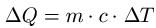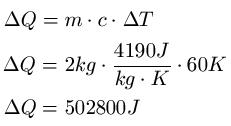# How is thermal energy transferred from fuels

## Thermal energy

We deal with thermal energy in this article. We deal with different transmission methods and also give suitable examples and formulas. This article belongs to the field of thermodynamics.The thermal energy - often also called thermal energy - is the energy that is stored in the disordered movement of the atoms or molecules of a substance. It is a state variable and is part of the inner energy. Thermal energy can be transferred between bodies. The formula for the transfer of heat energy may only be used if the physical state of the body does not change (reading tip: physical state). The formula for the calculation is then:Where:

• "ΔQ" is the change in thermal energy in joules [J]
• "m" is the mass in kilograms [kg]
• "c" is the specific heat capacity in joules per kilogram times Kelvin [J / (kg · K)]
• "ΔT" is the temperature change in Kelvin [K]

Example:

Two liters of water should be heated from 20 degrees to 80 degrees. What kind of heat is required for this

Solution: The amount of water can be specified directly as weight, since 2 liters of water correspond to a mass of 2kg, so m = 2kg. The specific heat capacity of water is taken from a table and is c = 4190J kg-1K-1. The temperature difference is 60 degrees Celsius, which also corresponds to 60 Kelvin.Left:

### Who's Online

We have 244 guests online